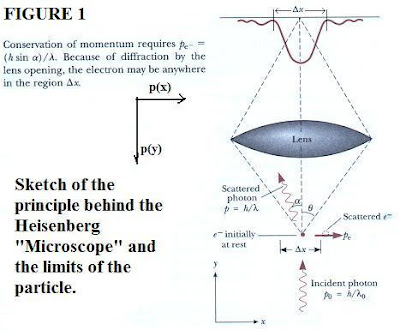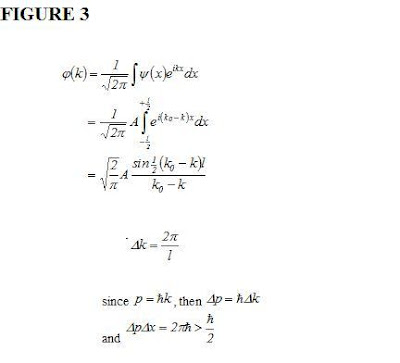## Monday, June 14, 2010

### More on the Heisenberg Microscope in Quantum MechanicsTwo blogs ago, I showed the Heisenberg microscope which is a theoretical device used to teach basic quantum mechanics. We visit that again, in order to become more clear on what exactly is meant by the "particle" and "wave" observational limits. The same drawing is reproduced, but now for convenience, I also show the momentum vectors, p(x) and p(y).

Particle approach:

Consider a measurement made to determine the instantaneous position of an electron by means of such a microscope. In such a measurement the electron must be illuminated, because it is actually the light quanta (photon) scattered by the electron that the observer sees. The resolving power of the microscope determines the ultimate accuracy with which the electron can be located.

The resolving power is known to be approximately (L/2 sin (theta)) - where L denotes the wavelength or the Greek letter lambda in the diagram. This is the wavelength of the scattered light and is the half angle subtended by the objective lens of the microscope. To be collected by the lens, the photon must be scattered through any angle ranging from (-theta) to theta .

Consequently, the electron’s momentum value values range from (h sin(theta)/L) to (-h sin(theta)/L) . Thus the uncertainty in x-component of the momentum, delta p(x), is given by:

delta(p(x)) = 2 h sin(theta)/ L

Hence: delta(p(x)) * delta (x) = h

in agreement with the Uncertainty principle.

The key in the particle approach, is that any scattered electron (or photon) will be describable by the de Broglie relation:

p = h / mv

where m is the mass of the particle, and v its velocity.

In the wave approach, the end result will simply be consonant with replacing the quantity (mv) by L the wavelength.

Wave approach:

Imagine that the shutter shown (top of diagram with delta (x) ) is open abruptly and then closed again, allowing a single particle to pass through. A wave function whose spatial dependence is of the form

A exp (iK_ox)

arrives from the left as shown (Fig. 2).

Call the associated wave function U(x), then its profile can be described:

U(x) = 0, for x < -l/2 U(x) = A exp (iK_ox) , for -l/2 <> l/2

so, the particle's position is localized between (-l/2) and (l/2). So, delta (x) ~ l.Then, to find the momentum wave function we simply integrate as shown in Fig. 3, leading to the result shown (bottom line) which is again in agreement with the Uncertainty Principle. Thus, the same device can be used to obtain uncertainty principles for particles and waves, in slightly differing limits.

Whether a particle or wave will be visible depends on the scale of delta(k) in relation to delta(x). The wavelength in general will be such that L ~ 1/k. I.e. as k retreats in size, L becomes larger.

Technically, the wave-particle duality is actually a reflection of the Principle of Complementarity in Quantum physics. usually expressed via the Poisson brackets (with non-commuting variables x, p):

[x, p] = -i h = -i h/ 2pi

where h is the Planck constant of action. If two variables a, b commute, then one has:

[a, b] = (a*b - b*a) = 0

if not, then: [a,b] = (a*b - b*a) = -1

and we say a and b are 'non-commuting'. (You may observe one aspect at any one time, but not the other).

In term's of Bohr's (Complementarity) Principle, the variables x (position) and p(momentum) are regarded as "mutually interfering observables". This is why only one can be obtained to precision, while you lose the other.

In another sense, one can think of approaching a particle in such way (or with such apparatus as the Heisenberg microscope) that it suddenly gets 'wavy' (on account of the scale of k in relation to dx). At that stage, as Bohm notes, the particle aspect vanishes and you have a wave.